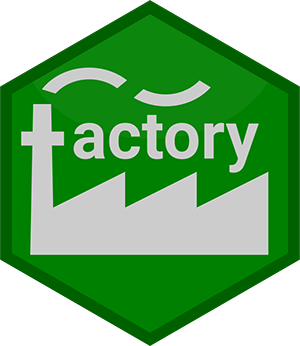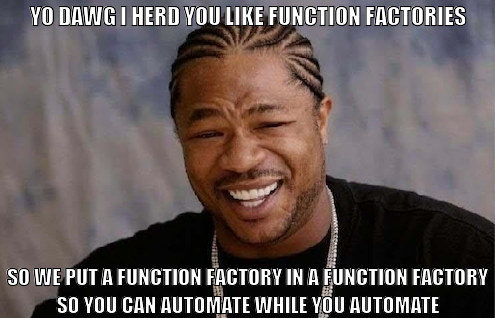# Introducing {factory}: Automate Your AutomationFunction factories are, in my opinion, one of the cooler concepts in programming. A function factory is a function that produces a function. A straightforward example function factory (from Advanced R (second edition) by Hadley Wickham) is a power function factory, which can be used to produce functions that raise their input to a specific power. You’d probably never actually use this exact factory, but it gets the general idea across:

power1 <- function(exp) {
function(x) {
x ^ exp
}
}

square <- power1(2)
cube <- power1(3)

square(3)
##  9
cube(3)
##  27

However, there’s a reason that particular factory is named power1 in Advanced R. That factory is fragile:

y <- 2
square <- power1(y)
y <- 3
square(2)
##  8

Since y changed before we called square, square didn’t work how we expected it to work. You can get around this by forceing the input to evaluate:

power2 <- function(exp) {
force(exp)
function(x) {
x ^ exp
}
}

y <- 2
square <- power2(y)
y <- 3
square(2)
##  4

That requirement makes function factories a bit more complicated to code. For an extreme example, see this pull request to the scales package, which took almost two years to get everything nailed down.

Even when you remember to force evaluation of variables inside the factory, the function produced by a factory isn’t quite what you might expect it to be:

square
## function(x) {
##     x ^ exp
##   }
## <environment: 0x00000000161e58a0>

Looking at that code, you can’t really tell what the function does. What is exp? How does the function know that it’s 2? To figure that out, you need to dig into the environment that’s displayed when you print square. It would be nicer if the output of a factory was a normal function that users could then adapt and work with like any other function.

The {rlang} package solves this problem by introducing the function new_function (as described in Advanced R by Hadley Wickham (2nd Edition), 19.7.4: Creating functions):

power3 <- function(exp) {
rlang::new_function(
rlang::exprs(x = ),
rlang::expr({
x ^ !!exp
}),
rlang::caller_env()
)
}

y <- 2
square <- power3(y)
y <- 3
square(2)
##  4
square
## function (x)
## {
##     x^2
## }

Now the resulting function looks like any other function… but we’ve just moved the complexity from the factory user to the factory author.

My goal with {factory} is to make factory creation easy for both the author and user.

library(factory)
power4 <- build_factory(
fun = function(x) {
x^exp
},
exp
# For the time being, you need to tell factory which arguments
# belong to the factory.
)

x <- 2
square <- power4(x)
x <- 3
square(2)
##  4
square
## function (x)
## {
##     x^2
## }

The function is as easy to write as in power1, and the resulting function is a “normal” function as in power3.

With help from Colin Fay, I’m currently working on adding an Rstudio addin to {factory}, to allow you to select some code (an instance of what you expect as output from a factory) and convert it into a factory. I also plan to add an addin to make it easy to use a factory while creating a package (a whole other can of worms), but that will wait for a future blog post.

Do you have any function factory use cases? Let me know here, or, more importantly, in a {factory} issue!

library(memer)
meme_get("YoDawg") %>%
meme_text_top(
txt = toupper("Yo dawg I herd you like function factories"),
size = 24
) %>%
meme_text_bottom(
txt = toupper(
paste(
"so we put a function factory in a function factory",
"so you can automate while you automate",
sep = "\n"
)
),
size = 22
)